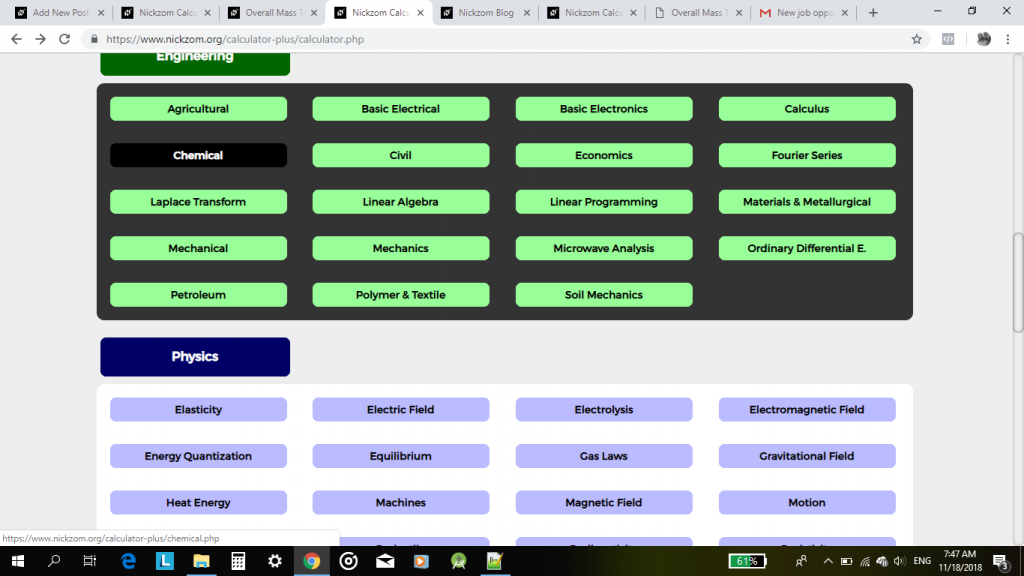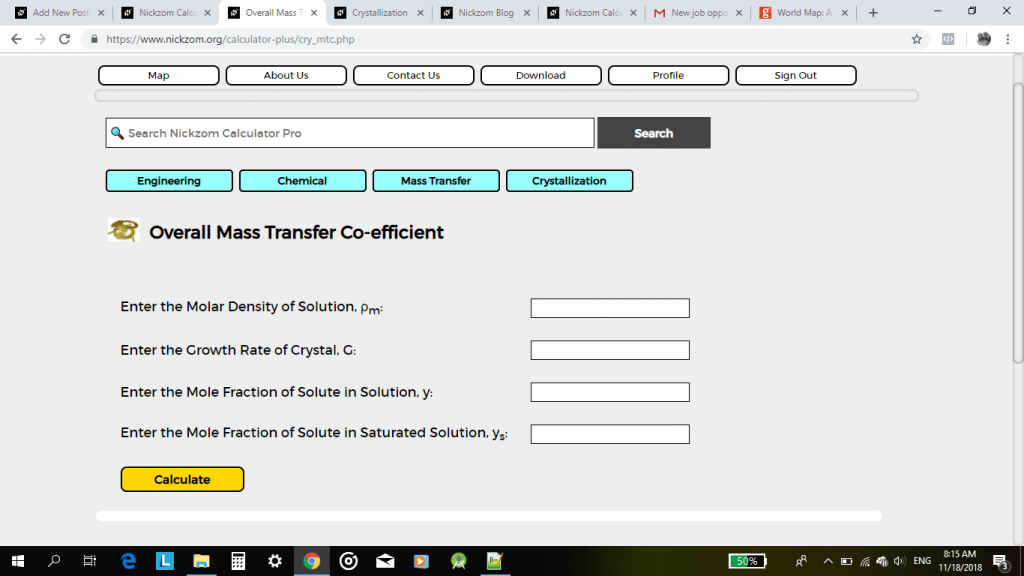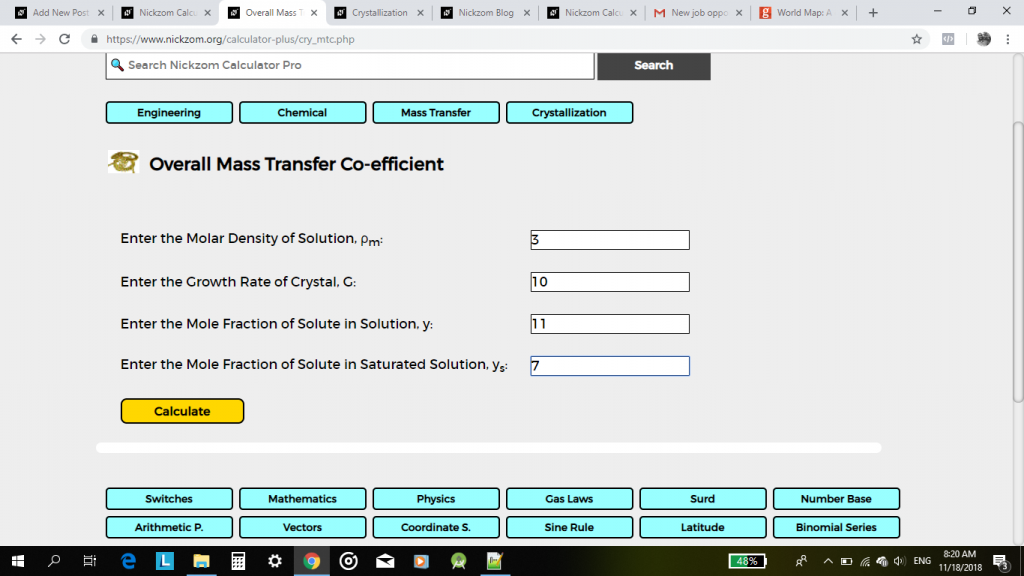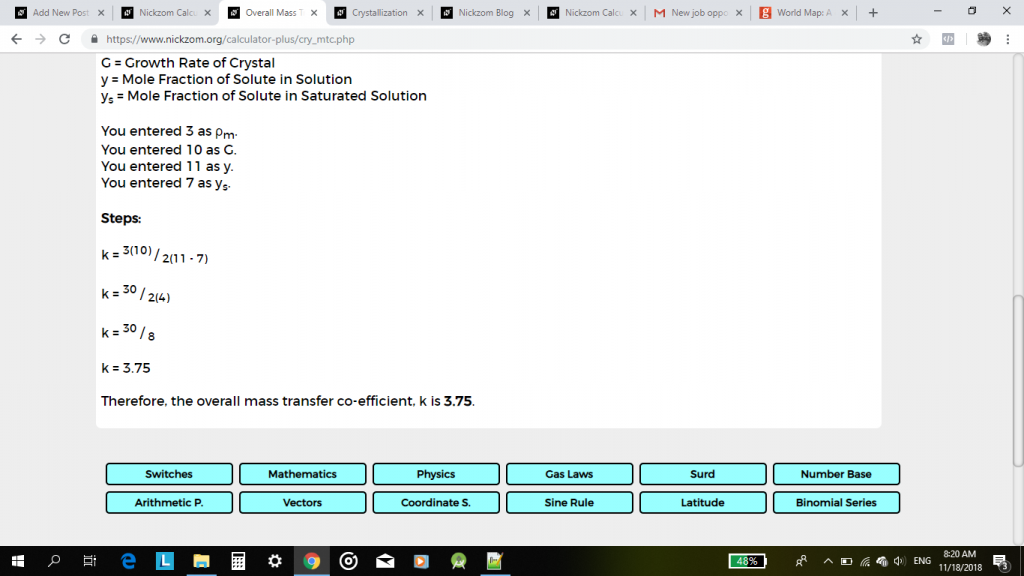# The Calculator Encyclopedia Calculates the Overall Mass Transfer Co-efficient in Crystallization for Chemical Engineering

Nickzom Calculator+ calculates virtually all the mathematical problems faced by an undergraduate of chemical engineering.

Today, we would be handling the obtaining of answers on overall mass transfer co-efficient which is under crystallization in Chemical engineering by Nickzom Calculator+.

One can access Nickzom Calculator+ (Professional Version) – The Calculator Encyclopedia via any of these channels:

First and foremost, if I want to get answers to my crystallization problem on Overall Mass Transfer Coefficient, I will proceed to my calculator map and click on Chemical (under Engineering Section).Once clicked, the Chemical engineering section is displayed for you with a few links to major topics that has calculations in Chemical engineering such as Fluid Mechanics, Mass Transfer and Heat Transfer.Mass Transfer has a bunch of links to various calculations but for the purpose of this topic we would focus on Crystallization.Now, click on crystallizationClick on Overall Mass Transfer Co-efficient,To obtain the overall mass transfer co-efficient, one needs to know the values of these parameters:

• Molar Density of Solution
• Growth Rate of Crystal
• Mole Fraction of Solute in Solution
• Mole Fraction of Solute in Saturated SolutionFinally, click on Calculate buttonThe answer is clearly yours with the formulas, parameters, workings and solution.